## Example Questions

2 Next →

### Example Question #11 : How To Find The Domain Of A Function

What is the domain of the following function?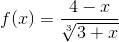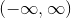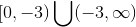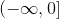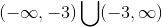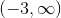Explanation:

The two potential concerns for domain in a standard function are negative numbers inside even-powered radicals, and dividing by zero. In this case, the radical we have is odd-powered, so having a negative result underneath the radical is fine. All we need to do, then, is avoid dividing by zero, which means avoiding a result which sums to zero under the radical. In this case, onlywill create this situation, so we must avoid it.

Thus, our domain is### Example Question #12 : How To Find The Domain Of A Function

Find the domain of the following function: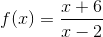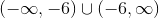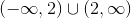Explanation:

To find the domain, you must find the values for which you can plug in for. Based on the given function, you know that you can not have a demoninator equal to. So, by setting the denominator equal to, you can find out the values that can not be in the domain, and then simply remove them from your set of numbers from negative infinity to positive infinity.

Thus,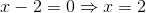Therefore, for our function,can not equalor it will be undefined. Thus, we get the set of numbers excluding positive.2 Next →